# RS Aggarwal Solutions for Class 8 Maths Chapter 18 - Area of a Trapezium and a Polygon Exercise 18B

Students can refer and download RS Aggarwal Solutions for Class 8 Maths Chapter 18- Exercise 18B, Area of a Trapezium and a Polygon from the links provided below. Our experts have solved the RS Aggarwal Solutions to ensure that the students are thorough with their basic concepts by practicing the solutions.

In Exercise 18B of RS Aggarwal Class 8 Maths, we know that the plots and fields are generally in the form of regular or irregular polygons. We find their areas by dividing them into triangles, rectangles, parallelograms and trapeziums.

## Download PDF of RS Aggarwal Solutions for Class 8 Maths Chapter 18- Area of a Trapezium and a Polygon Exercise 18B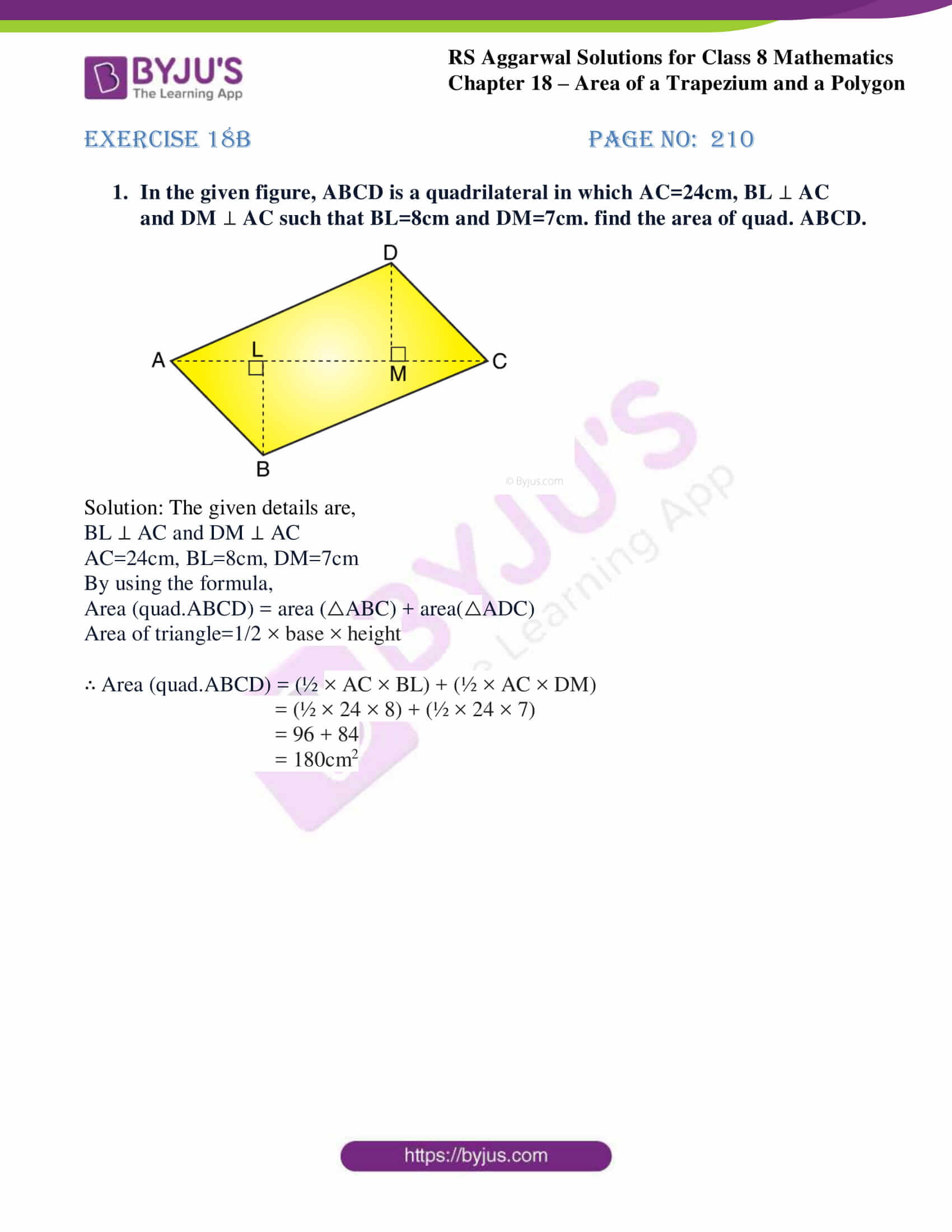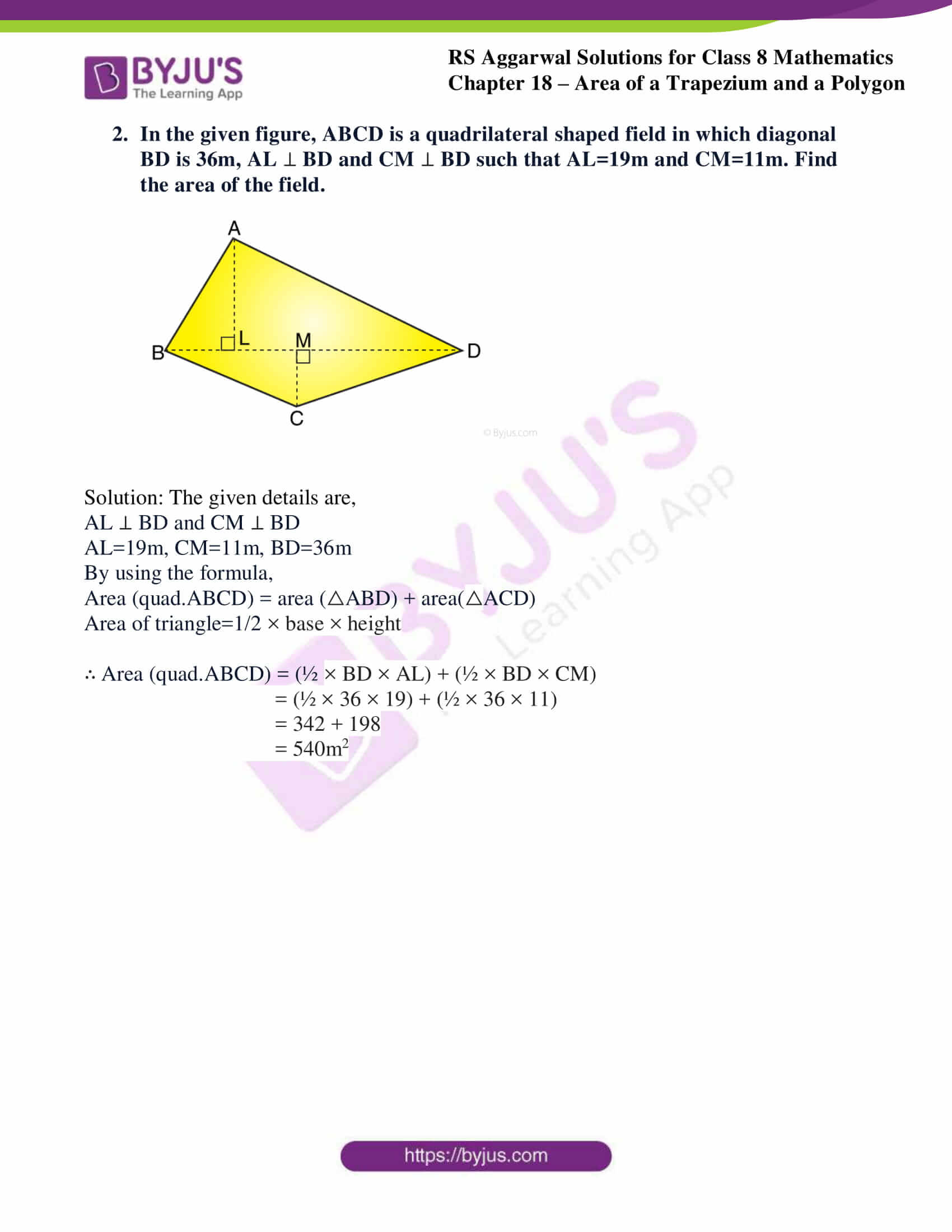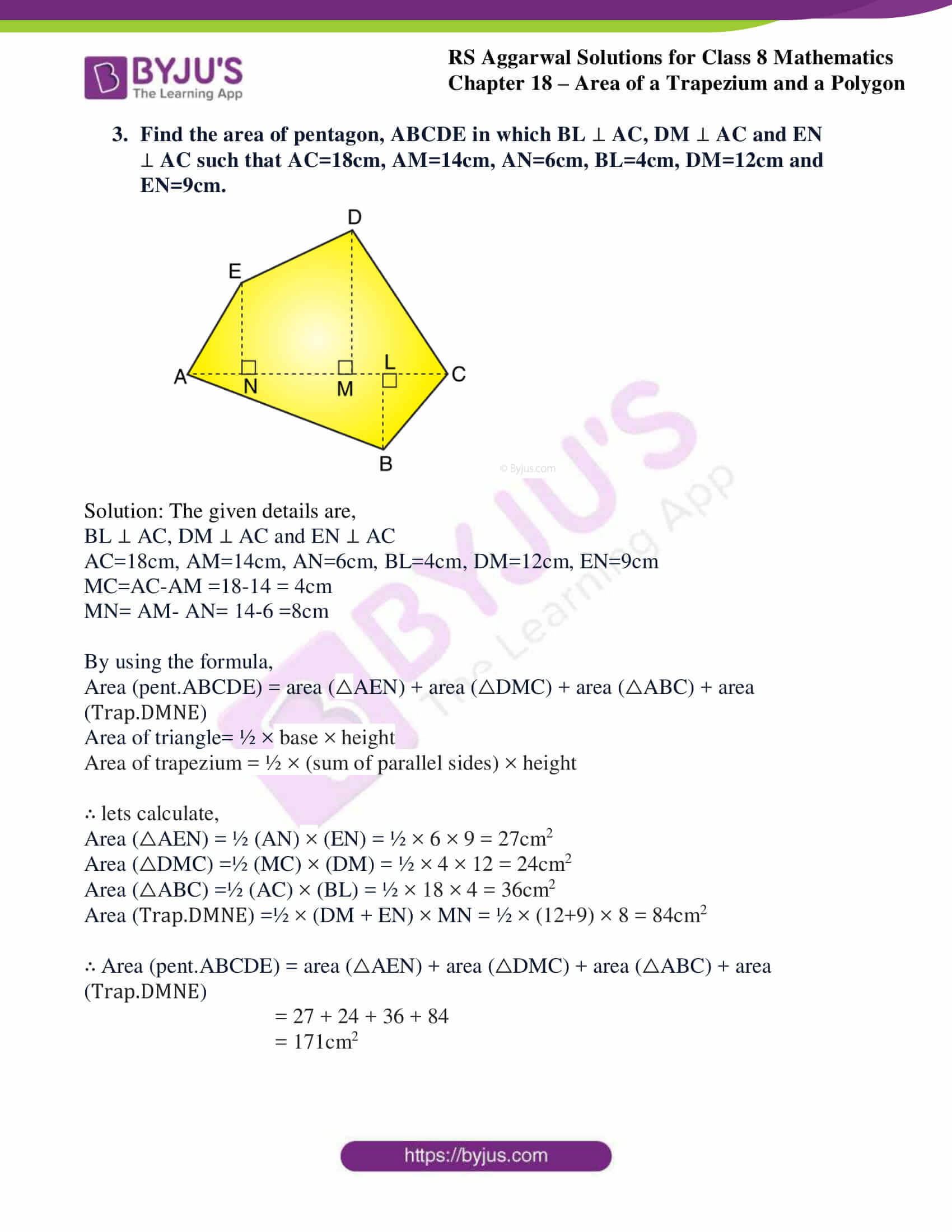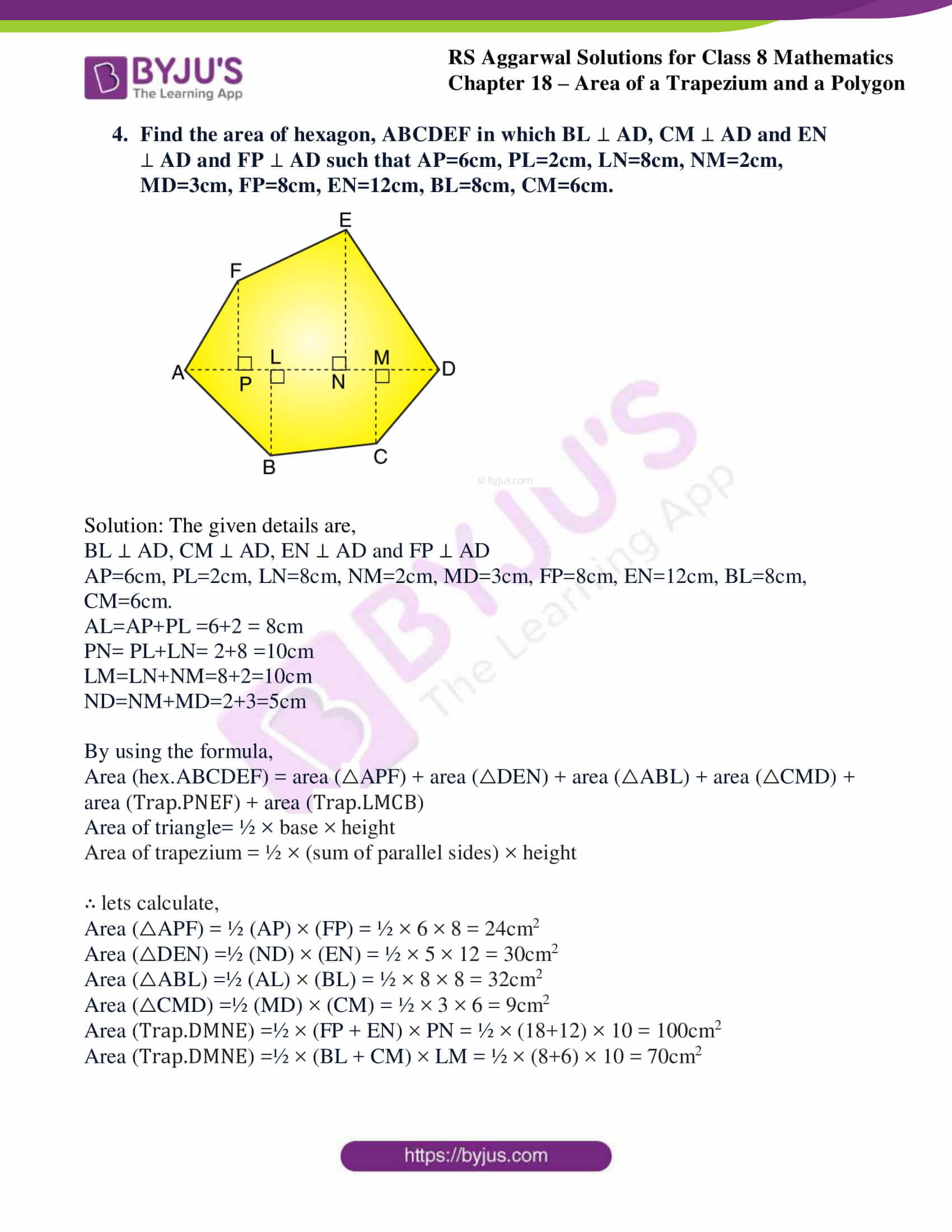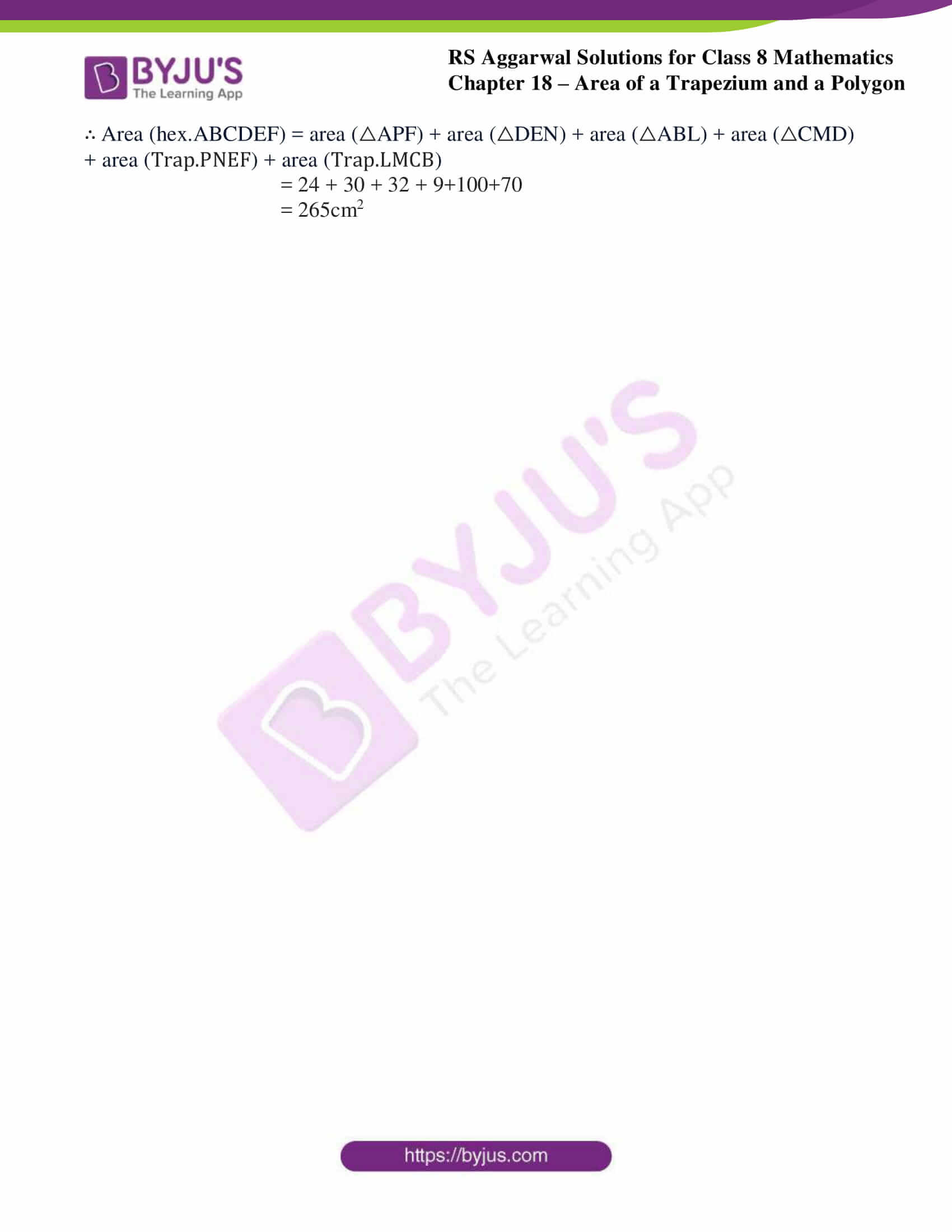### Access Answers to RS Aggarwal Solutions for Class 8 Maths Chapter 18- Area of a Trapezium and a Polygon Exercise 18B

1. In the given figure, ABCD is a quadrilateral in which AC=24cm, BL  AC and DM  AC such that BL=8cm and DM=7cm. find the area of quad. ABCD.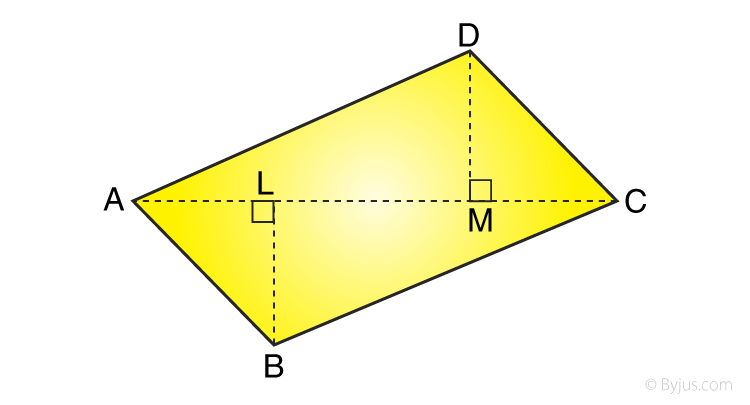Solution: The given details are,

BL ⊥ AC and DM ⊥ AC

AC=24cm, BL=8cm, DM=7cm

By using the formula,

Area of triangle=1/2 × base × height

∴ Area (quad.ABCD) = (½ × AC × BL) + (½ × AC × DM)

= (½ × 24 × 8) + (½ × 24 × 7)

= 96 + 84

= 180cm2

2. In the given figure, ABCD is a quadrilateral shaped field in which diagonal BD is 36m, AL  BD and CM  BD such that AL=19m and CM=11m. Find the area of the field.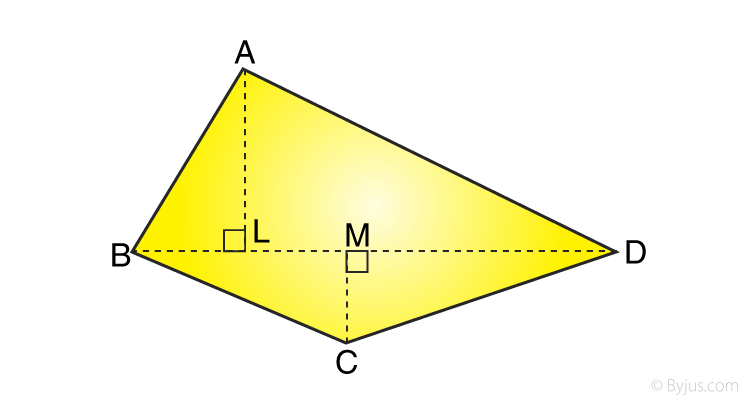Solution: The given details are,

AL ⊥ BD and CM ⊥ BD

AL=19m, CM=11m, BD=36m

By using the formula,

Area (quad.ABCD) = area (△ABD) + area(△ACD)

Area of triangle=1/2 × base × height

∴ Area (quad.ABCD) = (½ × BD × AL) + (½ × BD × CM)

= (½ × 36 × 19) + (½ × 36 × 11)

= 342 + 198

= 540m2

3. Find the area of pentagon, ABCDE in which BL  AC, DM  AC and EN  AC such that AC=18cm, AM=14cm, AN=6cm, BL=4cm, DM=12cm and EN=9cm.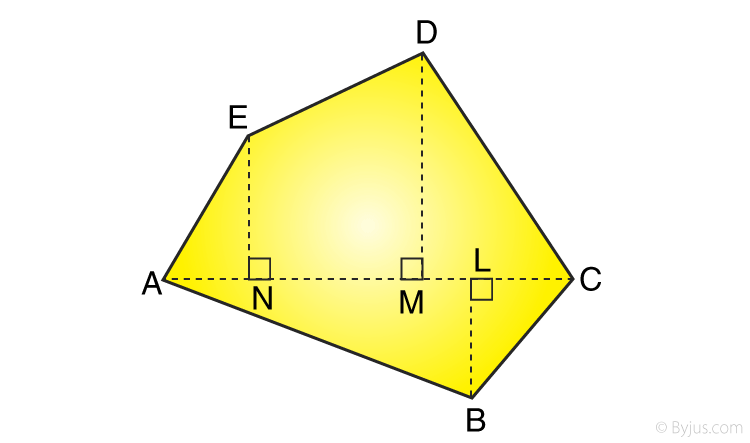Solution: The given details are,

BL ⊥ AC, DM ⊥ AC and EN ⊥ AC

AC=18cm, AM=14cm, AN=6cm, BL=4cm, DM=12cm, EN=9cm

MC=AC-AM =18-14 = 4cm

MN= AM- AN= 14-6 =8cm

By using the formula,

Area (pent.ABCDE) = area (△AEN) + area (△DMC) + area (△ABC) + area (Trap.DMNE)

Area of triangle= ½ × base × height

Area of trapezium = ½ × (sum of parallel sides) × height

∴ lets calculate,

Area (△AEN) = ½ (AN) × (EN) = ½ × 6 × 9 = 27cm2

Area (△DMC) =½ (MC) × (DM) = ½ × 4 × 12 = 24cm2

Area (△ABC) =½ (AC) × (BL) = ½ × 18 × 4 = 36cm2

Area (Trap.DMNE) =½ × (DM + EN) × MN = ½ × (12+9) × 8 = 84cm2

∴ Area (pent.ABCDE) = area (△AEN) + area (△DMC) + area (△ABC) + area (Trap.DMNE)

= 27 + 24 + 36 + 84

= 171cm2

4. Find the area of hexagon, ABCDEF in which BL  AD, CM  AD and EN  AD and FP  AD such that AP=6cm, PL=2cm, LN=8cm, NM=2cm, MD=3cm, FP=8cm, EN=12cm, BL=8cm, CM=6cm.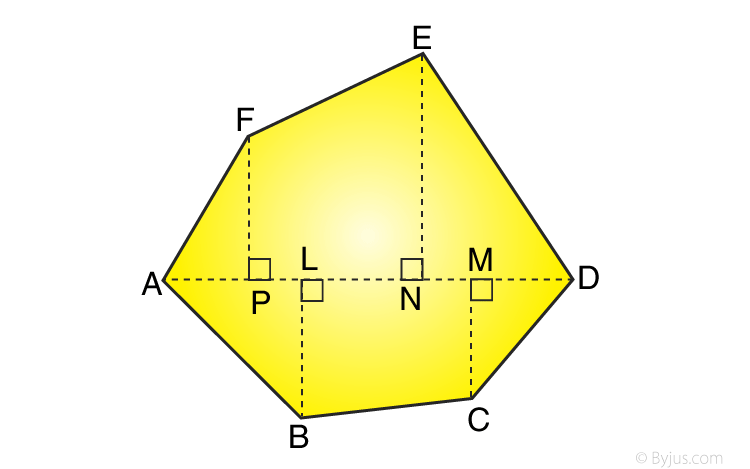Solution: The given details are,

AP=6cm, PL=2cm, LN=8cm, NM=2cm, MD=3cm, FP=8cm, EN=12cm, BL=8cm, CM=6cm.

AL=AP+PL =6+2 = 8cm

PN= PL+LN= 2+8 =10cm

LM=LN+NM=8+2=10cm

ND=NM+MD=2+3=5cm

By using the formula,

Area (hex.ABCDEF) = area (△APF) + area (△DEN) + area (△ABL) + area (△CMD) + area (Trap.PNEF) + area (Trap.LMCB)

Area of triangle= ½ × base × height

Area of trapezium = ½ × (sum of parallel sides) × height

∴ lets calculate,

Area (△APF) = ½ (AP) × (FP) = ½ × 6 × 8 = 24cm2

Area (△DEN) =½ (ND) × (EN) = ½ × 5 × 12 = 30cm2

Area (△ABL) =½ (AL) × (BL) = ½ × 8 × 8 = 32cm2

Area (△CMD) =½ (MD) × (CM) = ½ × 3 × 6 = 9cm2

Area (Trap.DMNE) =½ × (FP + EN) × PN = ½ × (18+12) × 10 = 100cm2

Area (Trap.DMNE) =½ × (BL + CM) × LM = ½ × (8+6) × 10 = 70cm2

∴ Area (hex.ABCDEF) = area (△APF) + area (△DEN) + area (△ABL) + area (△CMD) + area (Trap.PNEF) + area (Trap.LMCB)

= 24 + 30 + 32 + 9+100+70

= 265cm2

### Access other Exercises of RS Aggarwal Solutions for Class 8 Maths Chapter 18- Area of a Trapezium and a Polygon

Exercise 18A Solutions 12 Questions

Exercise 18C Solutions 5 Questions

## RS Aggarwal Solutions for Class 8 Maths Chapter 18- Area of a Trapezium and a Polygon Exercise 18B

Exercise 18B of RS Aggarwal Class 8, Area of a Trapezium and a Polygon. This exercise mainly deals with questions related to finding the area of a regular polygon and the area of an irregular polygon.

Students are suggested to practice the problems on a regular basis which will help them excel in their exams and increase their overall percentage. Practicing as many times as possible helps in building time management skills and also boosts the confidence level to achieve high marks.